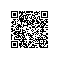# phalapi-入门篇5(数据库操作和Model层)## 1. 基于PDO的notorm进行的数据库操作

phalapi的数据库操作是使用的开源的notorm进行的,notorm是基于PDO链接数据库,在框架内部默认链接的是mysql数据库,如需修改链接其他数据库请修改 /PhalApi/PhalApi/DB/NotORM.php中的getPdo方法:

 $dsn = sprintf('mysql:dbname=%s;host=%s;port=%d',$dbCfg['name'],
isset($dbCfg['host']) ?$dbCfg['host'] : 'localhost',
isset($dbCfg['port']) ?$dbCfg['port'] : 3306
);


<?php
/**
* 数据库接口服务类
*/
class Api_DB extends PhalApi_Api{
public function getRules(){
return array(
'insert' => array(
'id'    => array('name' => 'id', 'require' => true, 'desc' => '用户Id'),
'name'  => array('name' => 'name', 'require' => true, 'desc' => '用户名称'),
'phone' => array('name' => 'phone', 'require' => true, 'desc' => '用户手机号码'),
),
'select' => array(
'id' => array('name' => 'id', 'require' => true, 'desc' => '用户Id'),
),
'update' => array(
'id'    => array('name' => 'id', 'require' => true, 'desc' => '用户Id'),
'name'  => array('name' => 'name', 'require' => true, 'desc' => '用户名称'),
'phone' => array('name' => 'phone', 'require' => true, 'desc' => '用户手机号码'),
),
'delete' => array(
'id' => array('name' => 'id', 'require' => true, 'desc' => '用户Id'),
),
);
}


### 1.1 insert接口

/**
* 新增表服务
* @return int id 新增列的Id
*/
public function insert(){
$data = array( //用数组构成需要插入键值一一对应 'id' =>$this->id,
'name'  => $this->name, 'phone' =>$this->phone,
);
$rs = DI()->notorm->user->insert($data);                  //执行数据库操作user代表的是表,返回结果是插入成功的值
return $rs['id']; //返回插入的id }  重要的是$rs = DI()->notorm->user->insert($data); 这段代码执行了sql语句,user是表名(这里的表名会加下在dbs中配置的表前缀组成一个完整的表名)我们试着运行一下http://localhost/Public/?service=DB.insert&name=miaomi&phone=13010001000&id=2会得到以下结果### 1.2 select接口 查询接口如下: /** * 查询 * @return array data 结果集 */ public function select(){$data   = array();
$data[] = DI()->notorm->user->select('name,phone')->where('id',$this->id)->fetch();
$data[] = DI()->notorm->user->select('name,phone')->where('id = ?',$this->id)->fetchAll();
$data[] = DI()->notorm->user->select('name,phone')->where('id != ?',$this->id)->fetchRows();

#### 1.2.3 fetch,fetchAll和fetchRows

fetch方法是获取单独的一条数据返回结果是不带下标的数组 ,fetchAll和fetchRows不同在于他们返回的是包含多条数据一个带下标的数组,可以看到在条件一样的情况下第一条和第二条查询出来的结果区别是第二条多了一个0的下标,从此可得到如果是确定返回结果只有一条优先使用fetch,如果是多条结果优先使用fetchAll和fetchRows.

fetchAll和fetchRows还提供了一个功能就是单独执行sql语句

$sql = 'select * from tbl_user where id = :id';$params = array(':id' => $this->id); //替换:id为请求参数的id DI()->notorm->user->queryAll($sql, $params); //或fetchRows($sql, $params)  这样就可以执行sql语句,包括一些复杂的查询sql可以使用此内方法执行(关联查询应当优先使用这种形式) ### 1.3 update接口 修改接口如下: /** * 修改 */ public function update(){$data = array(
'name'  => $this->name, 'phone' =>$this->phone,
);
$rs = DI()->notorm->user->where('id',$this->id)->update($data); if($rs === false){
}
}


}
}


http://localhost/Public/?service=DB.delete&id=2

return DI()->notorm->user->select('*')->where('id = ?', $userId)->fetch(); }  在内部直接封装数据库操作使用如下代码调用 $model = new Model_User();
$rs =$model->getByUserId($userId);  ### 2.2 框架自带的Model操作 当然这里介绍model的目的当然是解读一下phalapi内部提供的model操作 使用自带model操作只需要继承PhalApi_Model_NotORM 在实现如下方法 protected function getTableName($id) {
return 'user';
}


public function getByUserId($userId) { return$this->getORM()->select('*')->where('id = ?', $userId)->fetch(); } $this->getORM()相当于DI()->notorm->(getTableName中设置的表名)

public function select(){
$model = new Model_User(); return$model->getByUserId($this->id); }  可以获得以下结果在这里phalapi自带的model和传统的model对比起来区别在于,phalapi统一制定表名不会应为方法果断导致的表名写错的失误 另一方面phalapi自带的model提供了很多字基础操作,利用自动提示功能可以看到我们来再次改造一下selete接口使用model自带的方法 public function select(){$model = new Model_User();
return $model->get($this->id);
}


## 3. 总结使用钉钉扫一扫加入圈子
+ 订阅